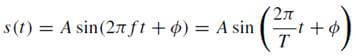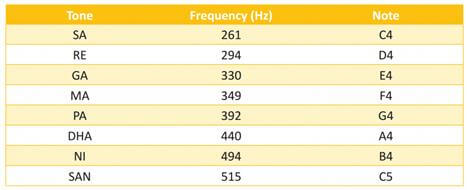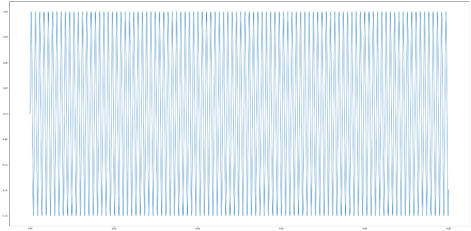# Project 3: Playing Sargam In Python

## Introduction

In this chapter, we will learn to make a musical instrument that can play Sargam in Python.

## Sargam

I was intrigued to play Sargam in Python. Found this simple audio package that plays numpy as music.

So, wanting to build Sargam notes on it, found a way to play Sargam and sharing the same here.

In the attachment, there is code that has two musical notes, one simple Sargam and one is a famous "naagin" tune.

So what are sound waves? A scientific definition is as follows.

Sound waves - The physical phenomenon we call sound is originated by air molecule oscillations due to the mechanical energy emitted by an acoustic source. The displacement s(t) with respect to the equilibrium position of each molecule can be modeled as a sine function.where,
• A – Amplitude
• T – Time period
• f – frequency measured in Hz
• f - phase
The Sargam has the following frequencies.The simpleaudio package of python makes it very interesting to create musical notes.

Step 1

Install simpleaudio
1. pip install simpleaudio
Step 2

Import these libraries
1. import numpy as np
2. import simpleaudio as sa
3. from itertools import repeat
Step 3

Create a dictionary of frequencies
1. sargamdict = {"sa":261,
2.              "re":294,
3.              "ga":330,
4.              "ma":349,
5.              "pa":392,
6.              "dha":440,
7.              "ni":494,
8.              "sa":515}
Step 4 - Define the play_audio function

Numpy arrays can be used to store audio but there are a few crucial requirements. If they are to store stereo audio, the array must have two columns since each column contains one channel of audio data. They must also have a signed 16-bit integer dtype and the sample amplitude values must consequently fall in the range of -32768 to 32767. Here is an example of a simple way to 'normalize' the audio (making it cover the whole amplitude rage but not exceeding it):
1. audio_array = audio_array.astype(np.int16)
And here is an example of converting it to the proper data type (note that this should always be done after normalization or other amplitude changes):
1. audio_array = audio_array.astype(np.int16)
2.
3. def play_audio(audio):# normalize to 16-bit range
4.     audio *= 32767 / np.max(np.abs(audio))
5.     # convert to 16-bit data
6.     audio = audio.astype(np.int16)
7.
8.     # start playback
9.     play_obj = sa.play_buffer(audio, 12, sample_rate)
10.
11.     # wait for playback to finish before exiting
12.     play_obj.wait_done()
Step 5

Get timesteps for each sample, T is note duration in seconds. For CD recordings, the industry standard is to store each audio sample as a 16-bit value, at 44100 samples per second.

sample_rate = 44100
1. T = 0.25
2. t = np.linspace(0, T, T * sample_rate, endpoint=False)
Step 6

Generate sine wave notes for sa re ga ma …
1. Sa_note = np.sin(sargamdict["sa"] * t * 3 * np.pi)
2. Re_note = np.sin(sargamdict["re"] * t * 3 * np.pi)
3. Ga_note = np.sin(sargamdict["ga"] * t * 3* np.pi)
4. Ma_note = np.sin(sargamdict["ma"] * t * 3 * np.pi)
5. Pa_note = np.sin(sargamdict["pa"] * t * 3 * np.pi)
6. Dha_note = np.sin(sargamdict["dha"] * t * 3 * np.pi)
7. Ni_note = np.sin(sargamdict["ni"] * t * 3 * np.pi)
8. Sa1_note = np.sin(sargamdict["sa1"] * t * 3 * np.pi)
Step 7

Create a pause note
1. get_pause  = lambda seconds: repeat(0, int(seconds * sample_rate))
2. pause_note=list(get_pause(0.01))
Step 8

Build the sargam
1. sargam = np.hstack((Sa_note,pause_note,Re_note,pause_note,Ga_note,
2.                    pause_note,Ma_note, pause_note,Pa_note, pause_note,
3.                     Dha_note, pause_note,Ni_note, pause_note, Sa1_note))
Step 9

and play it !!
1. play_audio(sargam)
2. play_audio(sargam[::-1])
In case you are interested to view the sin waves for each note, you can view using following
1. import matplotlib.pyplot as plt
2. %matplotlib inline
3. plt.figure(figsize=(40,20))
4. plt.plot(t, Sa_note);You can even save this numpy array as a .wav file using wavio library
1. pip install wavio
2. import wavio
3. fs = 44100
4. s2 = np.append(sargam,sargam[::-1])
5. wavio.write("pythonsargam.wav", s2, fs, scale=None, sampwidth=2)

## Conclusion

In the next chapter, you will learn to send mails using python smtplib.
Next » Project 4: Sending Email Through Python Console